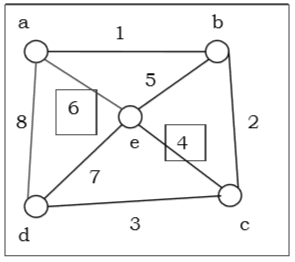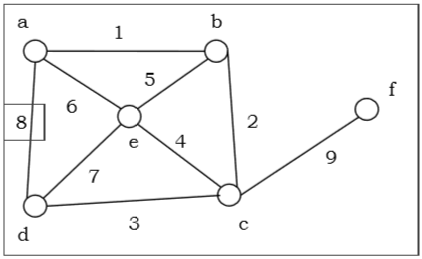# Hamiltonian Graphs

Hamiltonian graph - A connected graph G is called Hamiltonian graph if there is a cycle which includes every vertex of G and the cycle is called Hamiltonian cycle. Hamiltonian walk in graph G is a walk that passes through each vertex exactly once.

Dirac's Theorem - If G is a simple graph with n vertices, where n ≥ 3 If deg(v) ≥ {n}/{2} for each vertex v, then the graph G is Hamiltonian graph.

Ore's Theorem - If G is a simple graph with n vertices, where n ≥ 2 if deg(x) + deg(y) ≥ n for each pair of non-adjacent vertices x and y, then the graph G is Hamiltonian graph.In above example, sum of degree of a and c vertices is 6 and is greater than total vertices, 5 using Ore's theorem, it is an Hamiltonian Graph.

## Non-Hamiltonian GraphIn above example, sum of degree of a and f vertices is 4 and is less than total vertices, 4 using Ore's theorem, it is not an Hamiltonian Graph.